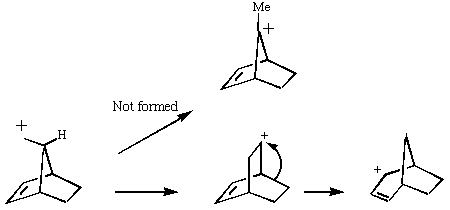The Stability of Carbonium Ions

Organic chemists are taught that carbonium ion stability is predominantly due to electronic factors such as whether they are primary, secondary, tertiary, allylic, benzylic etc. What is mentioned much less is that their relative stability is also very sensitive to their geometries, and in particular the angles of the three substituents at the carbon. We will use a technique known as Molecular Mechanics to predict the relative stabilities of the species shown below.

The only surprising result is that the MM method predicts that the tertiary carbonium ion is actually LESS stable than the preceeding primary ion, which is surprising to say the least. Clearly, electronic factors must be playing an important role as well. Suffice to say that even without ANY consideration of electronic factors, the MM2 method does not do too badly in its prediction of relative stabilities of this set of carbonium ions. This re-inforces the conclusion that bond angles in carbonium ions are just as important as substitution! Take a look for example at the angle contribution to the MM energies, which varies most. Also included are the semii-empirial (PM3) energies, which take into account lots of other factors, including effects such as hyper-conjugation, and p conjugation. In this particular system, the differences in these effects are only secondary in controlling the stability.stretch = 0.868 angle = 19.173 stretch bend = -0.672 dihedral = 6.497 improp torsion = 0.155 van der Waals = 2.663 electrostatics = -1.341 hydrogen bond = 0.000 The energy of the final structure is 27.342 kcal/mol. (Final QM heat of formation = 249.11 Kcal/mol) stretch = 0.447 angle = 18.758 stretch bend = -0.078 dihedral = 6.916 improp torsion = 1.124 van der Waals = 1.622 electrostatics = 0.000 hydrogen bond = 0.000 The energy of the final structure is 28.788 kcal/mol. (Final QM heat of formation = 232.62 Kcal/mol) stretch = 0.571 angle = 5.694 stretch bend = -0.027 dihedral = 7.179 improp torsion = 0.009 van der Waals = 5.678 electrostatics = -0.545 hydrogen bond = 0.000 The energy of the final structure is 18.559 kcal/mol. (Final QM heat of formation = 211.67 Kcal/mol) stretch = 0.512 angle = 3.439 stretch bend = -0.153 dihedral = 6.662 improp torsion = 0.002 van der Waals = 3.872 electrostatics = 0.683 hydrogen bond = 0.000 The energy of the final structure is 15.017 kcal/mol. (Final QM heat of formation = 195.79 Kcal/mol)